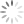Loading, please wait...# loops statement in C Programming

Aug 21, 2019 c programming, loop, 2341 Views

Loops are the control statements in c programming also used to perform looping operations until the given condition is true. Control comes out of the loop statements once the condition becomes false.

Types of loop control statements in C:

There are 3 types of loop control statements in C language. They are

1) For

2) While

3) do-while

the syntax for each C loop control statement is given in the below table with description.

 S. no. Loop name syntax Description 1 for For (exp1; exp2; exp3) {statements;} Where, exp1 - variable initialization (example: i=0, j=2, k=3) exp- 2 - condition checking (example i>5,j<3, k=3) exp – 3 increment/decrement (example: ++i, j-, ++k) 2 while While {condition} {statements;} Where, condition might be a>, j<10 3 do while Do{statements;} while(condition); Where, condition might be a>5, j<10

### Example program (for loop) in C:

In for loop control statement, the loop is executed until the condition becomes false.

``````#include<stdio.h>
int main()
{
int i;
for(i=0;i<10;i++)
{
printf(“%d”,i);
}
}``````

output:

``0 1 2 3 4 5 6 7 8 9``

### Example program (while loop) in C:

In while loop control statement, the loop is executed until the condition becomes false.

``````#include<stdio.h>
int main()
{
int i=3;
while(i<10)
{
printf(“%d\n”,i);
i++;
}
}``````

output:

``3 4 5 6 7 8 9``

### Example program (do while loop) in C:

In do while loop control statement, while loop is executed irrespective of the condition for the first time. Then 2nd time onwards, the loop is executed until the condition becomes false.

``````#include <stdio.h>
int main()
{
int i=1;
do
{
printf(“value of i is %d\n”,i);
i++;
}while (i<4 && i>=2);
}``````

Output:

``````Value of i is 1

Value of i is 2

Value of i is 3

Value of i is 4​``````